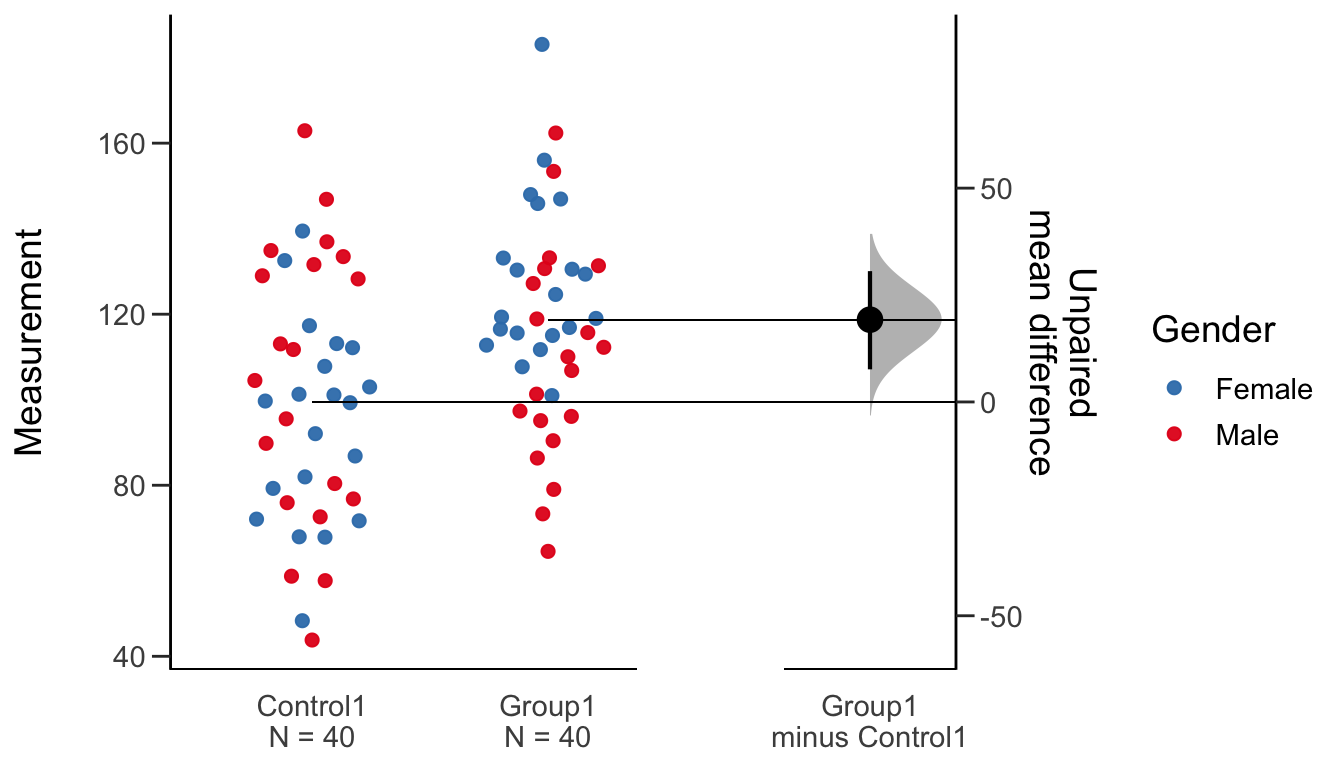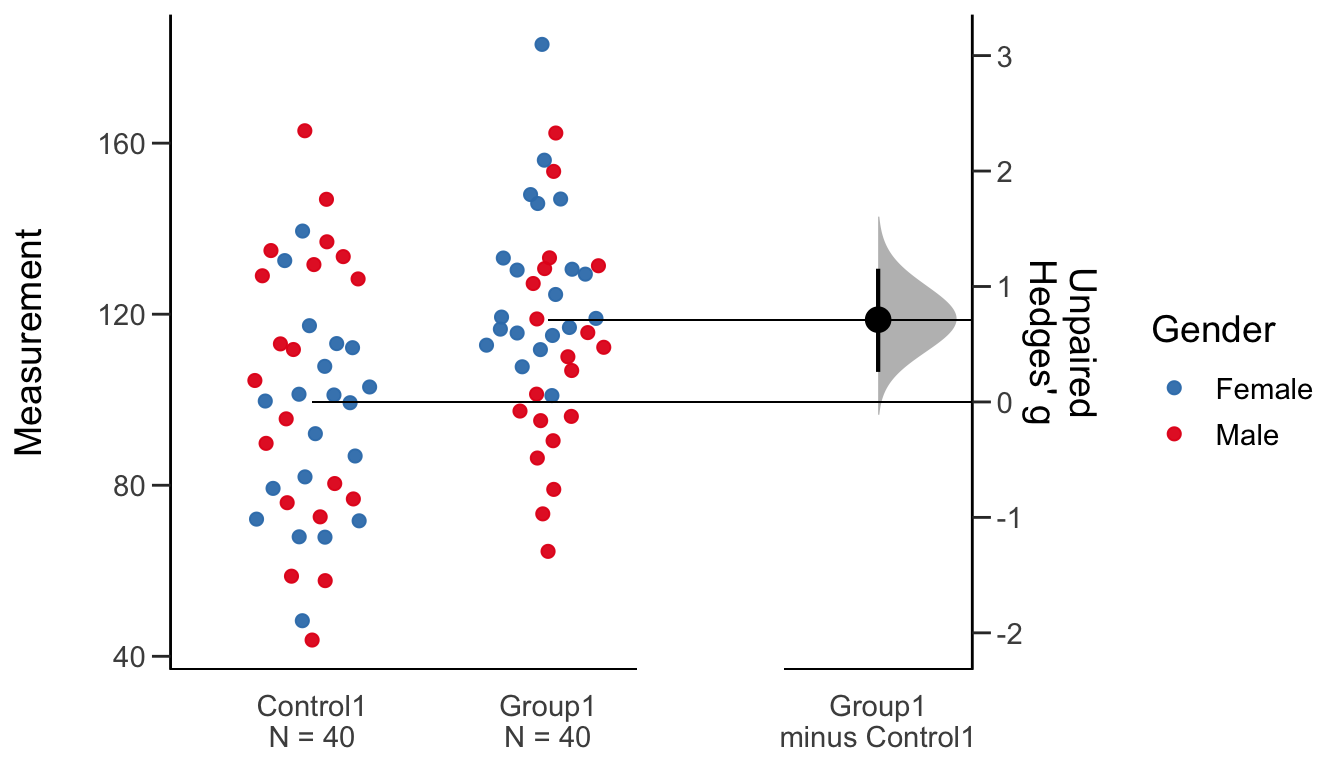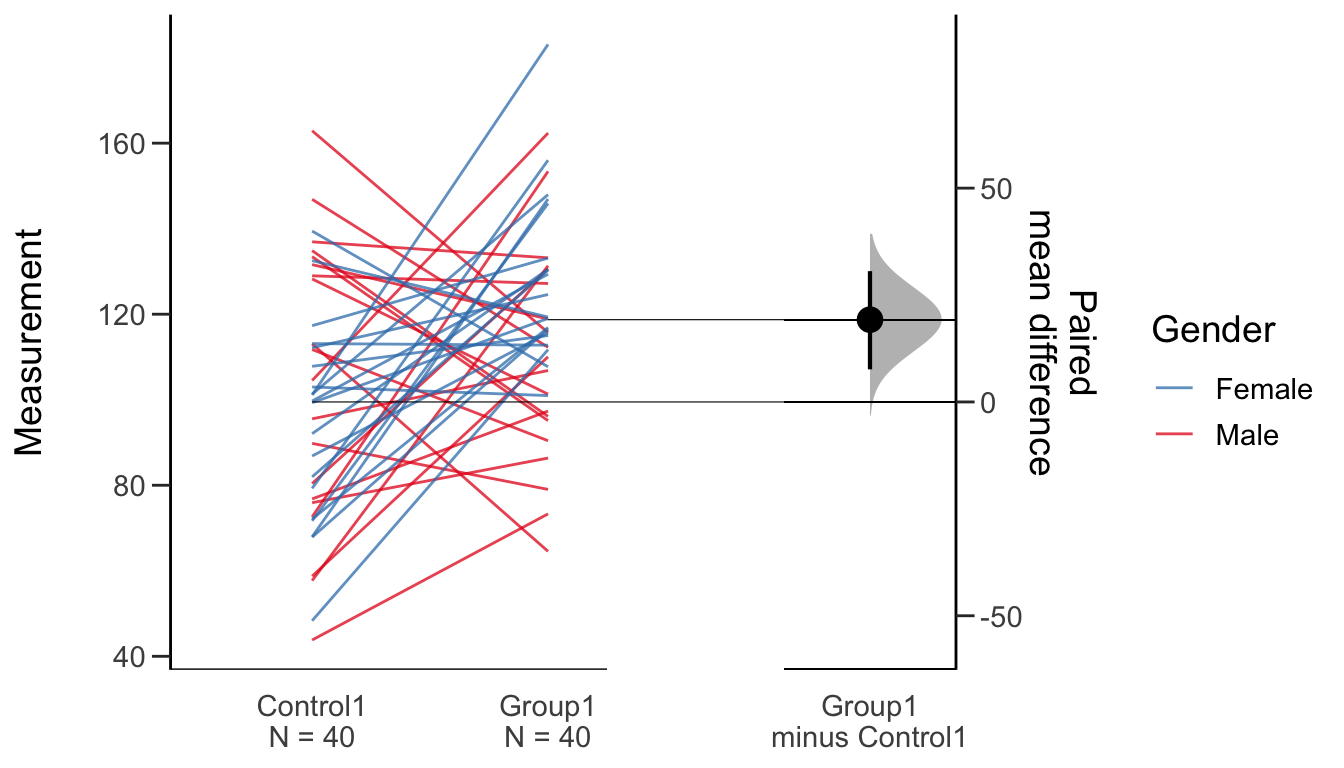# Create Data

For this vignette, we will create and use a synthetic dataset.

``````library(dplyr)

set.seed(54321)

N = 40
c1 <- rnorm(N, mean = 100, sd = 25)
c2 <- rnorm(N, mean = 100, sd = 50)
g1 <- rnorm(N, mean = 120, sd = 25)
g2 <- rnorm(N, mean = 80, sd = 50)
g3 <- rnorm(N, mean = 100, sd = 12)
g4 <- rnorm(N, mean = 100, sd = 50)
gender <- c(rep('Male', N/2), rep('Female', N/2))
dummy <- rep("Dummy", N)
id <- 1: N

wide.data <-
tibble::tibble(
Control1 = c1, Control2 = c2,
Group1 = g1, Group2 = g2, Group3 = g3, Group4 = g4,
Dummy = dummy,
Gender = gender, ID = id)

my.data   <-
wide.data %>%
tidyr::gather(key = Group, value = Measurement, -ID, -Gender, -Dummy)

``````## # A tibble: 6 x 5
##   Dummy Gender    ID Group    Measurement
##   <chr> <chr>  <int> <chr>          <dbl>
## 1 Dummy Male       1 Control1        95.5
## 2 Dummy Male       2 Control1        76.8
## 3 Dummy Male       3 Control1        80.4
## 4 Dummy Male       4 Control1        58.7
## 5 Dummy Male       5 Control1        89.8
## 6 Dummy Male       6 Control1        72.6``````

This dataset is a tidy dataset, where each observation (datapoint) is a row, and each variable (or associated metadata) is a column. `dabestr` requires that data be in this form, as do other popular R packages for data visualization and analysis.

# The Gardner-Altman Two Group Estimation Plot

## Unpaired

The `dabest` function is the main workhorse of the `dabestr` package. To create a two-group estimation plot (aka a Gardner-Altman plot), we must first specify the following:

• the `x` and `y` columns,
• whether the comparison is `paired = TRUE` or `paired = FALSE`,
• and the groups to be compared via `idx`.
``library(dabestr)``
``## Loading required package: magrittr``
``````two.group.unpaired <-
my.data %>%
dabest(Group, Measurement,
# The idx below passes "Control" as the control group,
# and "Group1" as the test group. The mean difference
# will be computed as mean(Group1) - mean(Control1).
idx = c("Control1", "Group1"),
paired = FALSE)

# Calling the object automatically prints out a summary.
two.group.unpaired ``````
``````## dabestr (Data Analysis with Bootstrap Estimation in R) v0.3.0
## =============================================================
##
## Good morning!
## The current time is 11:27 AM on Monday July 13, 2020.
##
## Dataset    :  .
## The first five rows are:
## # A tibble: 5 x 5
##   Dummy Gender    ID Group    Measurement
##   <chr> <chr>  <int> <fct>          <dbl>
## 1 Dummy Male       1 Control1        95.5
## 2 Dummy Male       2 Control1        76.8
## 3 Dummy Male       3 Control1        80.4
## 4 Dummy Male       4 Control1        58.7
## 5 Dummy Male       5 Control1        89.8
##
## X Variable :  Group
## Y Variable :  Measurement
##
## Effect sizes(s) will be computed for:
##   1. Group1 minus Control1``````

To compute the mean difference between `Group1` and `Control1`, we apply the `mean_diff()` function to the `dabest` object created above.

``````two.group.unpaired.meandiff <- mean_diff(two.group.unpaired)

# Calling the above object produces a textual summary of the computed effect size.
two.group.unpaired.meandiff``````
``````## dabestr (Data Analysis with Bootstrap Estimation in R) v0.3.0
## =============================================================
##
## Good morning!
## The current time is 11:27 AM on Monday July 13, 2020.
##
## Dataset    :  .
## X Variable :  Group
## Y Variable :  Measurement
##
## Unpaired mean difference of Group1 (n = 40) minus Control1 (n = 40)
##  19.2 [95CI  7.62; 30.6]
##
##
## 5000 bootstrap resamples.
## All confidence intervals are bias-corrected and accelerated.``````

As of `dabest` v0.3.0, there are five effect sizes available:

• The mean difference, given by `mean_diff()`.
• The median difference, given by `median_diff()`.
• Cohen’s d, given by `cohens_d()`.
• Hedges’ g, given by `hedges_g()`.
• Cliff’s delta, given by `cliffs_delta()`.

To create a two-group estimation plot (aka a Gardner-Altman plot) from this data, simply use `plot(dabest_effsize.object)`.

``plot(two.group.unpaired.meandiff, color.column = Gender)``This is known as a Gardner-Altman estimation plot, after Martin J. Gardner and Douglas Altman who were the first to publish it in 1986.

The key features of the Gardner-Altman estimation plot are:

1. All data points are plotted.
2. The mean difference (the effect size) and its 95% confidence interval (95% CI) is displayed as a point estimate and vertical bar respectively, on a separate but aligned axes.

The estimation plot produced by `dabest` differs from the one first introduced by Gardner and Altman in one important aspect. `dabest` derives the 95% CI through nonparametric bootstrap resampling. This enables visualization of the confidence interval as a graded sampling distribution.

The 95% CI presented is bias-corrected and accelerated (ie. a BCa bootstrap). You can read more about bootstrap resampling and BCa correction here.

You can also obtain Gardner-Altman plots for the median difference, Cohen’s d, and Hedges’ g effect sizes. Below we demonstrate how to obtain one for the Hedges’ g of the loaded `two.group.unpaired` dataset.

``two.group.unpaired %>% hedges_g() %>% plot(color.column = Gender)``## Paired

If you have paired or repeated observations, you must specify the `id.col`, a column in the data that indicates the identity of each paired observation. This will produce a Tufte slopegraph instead of a swarmplot.

``````two.group.paired <-
my.data %>%
dabest(Group, Measurement,
idx = c("Control1", "Group1"),
paired = TRUE, id.col = ID)

# The summary indicates this is a paired comparison.
two.group.paired``````
``````## dabestr (Data Analysis with Bootstrap Estimation in R) v0.3.0
## =============================================================
##
## Good morning!
## The current time is 11:27 AM on Monday July 13, 2020.
##
## Dataset    :  .
## The first five rows are:
## # A tibble: 5 x 5
##   Dummy Gender    ID Group    Measurement
##   <chr> <chr>  <int> <fct>          <dbl>
## 1 Dummy Male       1 Control1        95.5
## 2 Dummy Male       2 Control1        76.8
## 3 Dummy Male       3 Control1        80.4
## 4 Dummy Male       4 Control1        58.7
## 5 Dummy Male       5 Control1        89.8
##
## X Variable :  Group
## Y Variable :  Measurement
##
## Paired effect size(s) will be computed for:
##   1. Group1 minus Control1``````
``````# Create a paired plot.
two.group.paired %>%
mean_diff() %>%
plot(color.column = Gender)``````# The Cummings estimation plot

## Multi-two group

To create a multi-two group plot, one will need to specify a list, with each element of the list corresponding to the each two-group comparison.

``````multi.two.group.unpaired <-
my.data %>%
dabest(Group, Measurement,
idx = list(c("Control1", "Group1"),
c("Control2", "Group2")),
paired = FALSE)

# Compute the mean difference.
multi.two.group.unpaired.meandiff <- mean_diff(multi.two.group.unpaired)

# Create a multi-two group plot.
multi.two.group.unpaired.meandiff %>%
plot(color.column = Gender)``````